### Home > AC > Chapter 7 > Lesson 7.3.3 > Problem7-110

7-110.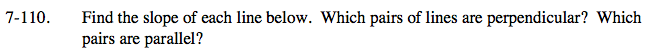Slopes that are opposite reciprocals of each other are perpendicular, while parallel lines share the same slope.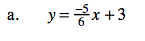Slope is given in the equation.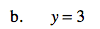y = 3 is a horizontal line, thus its slope is zero.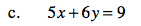Rearrange the equation into the y = mx + b form to identify the slope.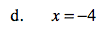x = −4 is a vertical line, thus its slope is undefined.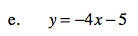Slope is given.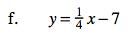Slope is given.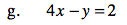Rearrange the equation into the y = mx + b form to identify the slope.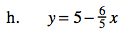Rearrange slightly and identify the slope.

An example of a pair of parallel lines is the line from part (a) and the line from part (c), while an example of a pair of perpendicular
lines is the line from part (b) and the line from part (d). Knowing this information, can you find your own examples?